December 7, 2021

# Class 10 Maths Chapter 8 Introduction To Trigonometry

Class 10 maths chapter 8 introduction to trigonometry is a totally new concept and is also considered as one of the difficult topics of class Xth maths. Since students see trigonometry for the first time, therefore, it’s natural to not understand it on the first attempt.

This may be your first time encountering trigonometry, but mind you, it is an integral part of mathematics and you will have to deal with it for the rest of your life if you are planning to take maths in higher classes. So, it’s best to clear all your doubts about this chapter.

In this chapter, we will mostly study the right-angled triangle and the relationship between the angles and sides of a right-angle triangle.

## Class 10 Maths Chapter 8 Introduction To Trigonometry

As usual, we will first understand the basic terminology for trigonometry so that we can learn things more effectively and easily.

What is trigonometry?

Trigonometry is a combination of 3 Greek words i.e ‘tri’ meaning ‘three’, ‘gon’ meaning ‘sides’, and ‘metron’ meaning ‘measure’. In simple words, trigonometry is the study of relationships between the sides and angles of a triangle.

As mentioned above in this article, trigonometry is all about triangles, and this is why the Pythagoras theorem is used extensively in this chapter. So, make absolutely sure that you understand this theorem in detail.

# Trigonometric Ratios

Class 10 maths chapter 8 Introduction to trigonometry: Trigonometric ratios
Trigonometric ratios Their reciprocal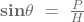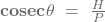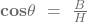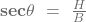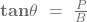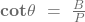In this table P = perpendicular, B = base, H = hypotenuse, θ = angle with respect to which the trigonometric ratios will be calculated.

Trigonometric ratios are simply the relationship between the sides and angles of a triangle (all the trigonometric ratios are given above in the table). We know that a right-angled triangle has a perpendicular, base, and a hypotenuse. The perpendicular along with the base makes a 90° angle this is why the triangle is called a right-angled triangle.

While calculating trigonometric ratios the important thing to notice is the angle with respect to which they will be calculated. Let’s understand it with an example.

Suppose there is a right-angled triangle ABC, if we want to calculate the trigonometric ratios with respect to angle A, then side AB will be base, BC will be perpendicular, and AC will be the hypotenuse. So, the trigonometric ratios will be: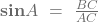,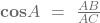,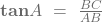,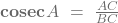,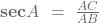,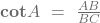Now, let’s assume that we want to calculate the same trigonometric ratios for the same triangle, but, this time with respect to angle C. You might be thinking that it will be the same as above, but no, the base and perpendicular will change here. As mentioned above, the angle is the key point here. Base and perpendicular will change according to angle.

So, if we are taking C as angle, then AB will now be perpendicular, CB will become base, and CA will remain hypotenuse. The trigonometric ratios will now be: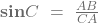,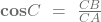,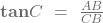,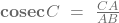,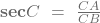,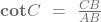The trick to find which side will be what while calculating the trigonometric ratios is simple. The side opposite to the angle will be perpendicular, and the side adjacent to the angle will become the base.

### Class 10 Maths Chapter 8 Introduction To Trigonometry

They say ‘beginning is the hardest part of any quest’, so we can say that we have completed the hardest part of the class 10 maths chapter 8 introduction to trigonometry. Now let’s move on to conquer the other topics of this chapter and learn more about them.

# Trigonometric ratios of some specific angles

Mathematicians have made things easy for us by giving the trigonometric ratios of some specific angles i.e 0º, 30º, 45º, and 90º.

Class 10 maths chapter 8 introduction to trigonometry: trigonometric ratios for some specific angles
∠A 30º 45º 90º
sin A 0 ½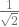1
cos A 1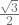0
tan A 0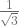1 not defined
cosec A not defined 2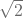1
sec A 1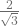not defined
cot A not defined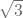1 0

To solve a question based on the above given specific trigonometric angles, all you have to do is put in the values of respective angles as given in the above table.

# Trigonometric ratios of complementary angles

To understand this topic we must first be aware of complementary angles. When the sum of two angles is 90° they are said to be complementary angles. Following are the trigonometric ratios of complementary angles:

Class 10 maths chapter 8 introduction to trigonometry:  complementary angles
sin (90°– A) = cos A cos (90°– A) = sin A
tan (90°– A) = cot A cot (90°– A) = tan A
sec (90°– A) = cosec A cosec (90°– A) = sec A

#### Class 10 Maths Chapter 8 Introduction To Trigonometry

At the beginning of this chapter we learned the trigonometric ratios, then we studied the values of some specific angles, then we came to know about the complementary angles, now for the final part of this chapter, we will learn some trigonometric identities. But first, we must know what is meant by identity in mathematics.

what is identity in Maths?

An identity is an equation that will always remain true irrespective of the values that will be replaced in it. In simple words, identity is a formula.

# Trigonometric identities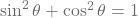With this identity we can derive 2 more identities i.e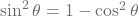and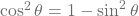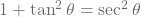Again with this identity two more identities can be derived i.e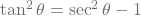and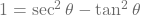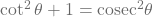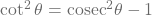,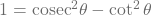So, these are all the trigonometric identities based on which questions will be asked in the board exam. Let’s now take a look at the blueprint of class 10 maths chapter 8 introduction to trigonometry.

Class 10 maths chapter 8 introduction to trigonometry blueprint
1 Marker 2 question
2 Marker 1 question
Total questions 4
Total marks 4

First of all, let’s make one thing clear that this blueprint is temporary meaning that it is subjected to change. So, for now, 4 questions will be asked in the board exam for this chapter. 2 questions of 1 mark and 1 question 2 marks.